# Samacheer Kalvi 6th Maths Solutions Term 1 Chapter 4 Geometry Ex 4.2## Tamilnadu Samacheer Kalvi 6th Maths Solutions Term 1 Chapter 4 Geometry Ex 4.2

Question 1.
Use any number of the given dots to make different angles.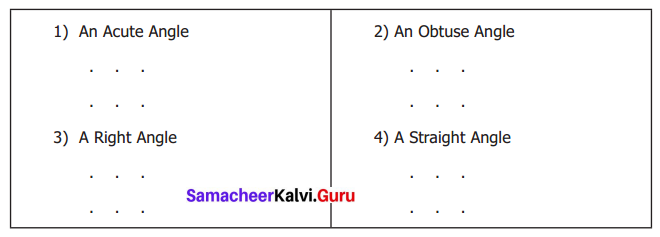Solution: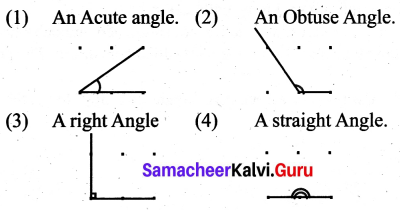Question 2.
Name the vertex and sides that form each angle.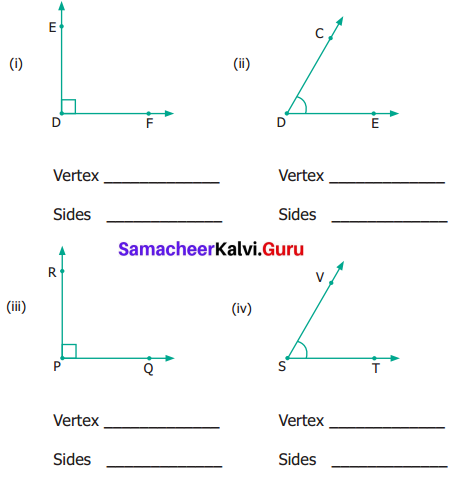Solution: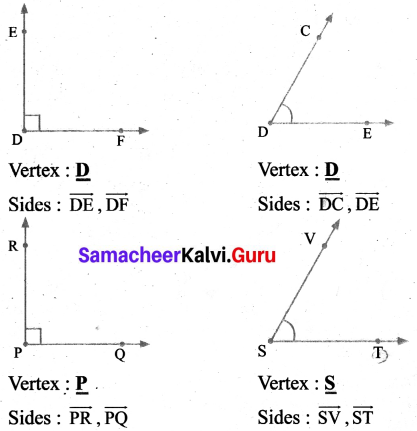Question 3.
Pick out the Right angles from the given figures.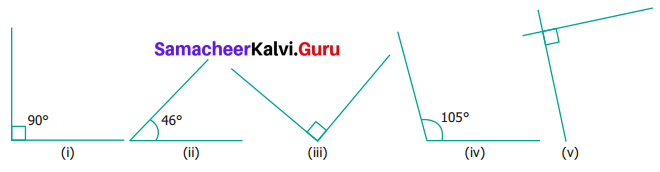Solution:
(i), (iii) and (v) are Right Angles.

Question 4.
Pick out the Acute angles from the given figures.Solution:
(i), (iii) and (iv) are the Acute Angles.

Question 5.
Pick out the Obtuse Angles from the given figures.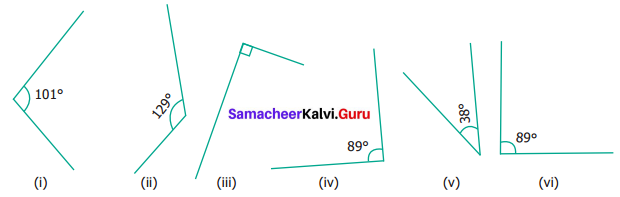Solution:
(i) and (ii) are the Obtuse Angles.Question 6.
Name the angle in each figure given below in all the possible ways.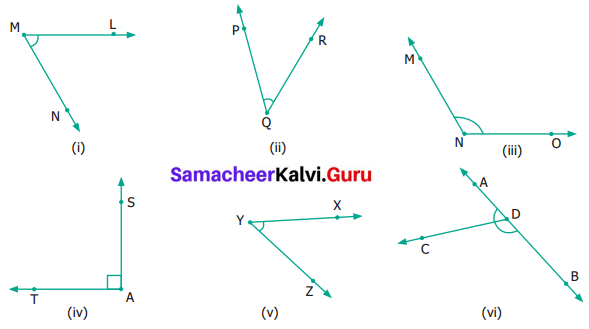Solution:
(i) ∠M or ∠LMN or ∠NML
(ii) ∠Q or ∠PQR or ∠RQP
(iii) ∠N or ∠MNO or ∠ONM
(iv) ∠A or ∠TAS or ∠SAT
(v) ∠Y or ∠XYZ or ∠ZYX
(vi) There are 3 angles in (vi)

• ∠ CDB or ∠BDC
• ∠D or ∠ADB or ∠BDA

Question 7.
Say True or False.

1. 20° and 70° are complementary.
2. 88° and 12° are complementary.
3. 80° and 180° are supplementary.
4. 0° and 180° are supplementary.

Solution:

1. True
2. False
3. False
4. True

Question 8.
Draw and label each of the angles
(i) ∠NAS = 90°
(ii) ∠BLG = 35°
(iii) ∠SMC = 145°
Solution: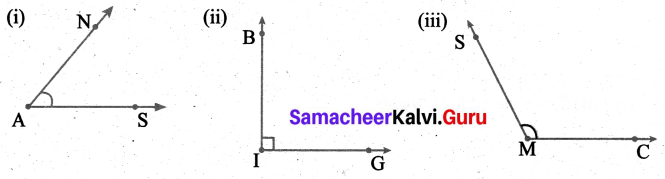Question 9.
Identify the types of angles shown by the hands of the given clock.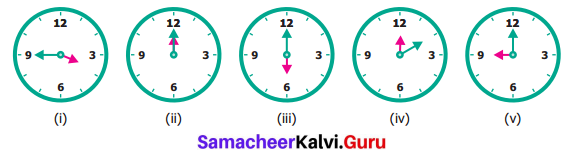Solution:
(i) Obtuse Angle.
(ii) Zero Angle.
(iii) Straight Angle.
(iv) Acute Angle.
(v) Right Angle.

Question 10.
Find the supplementary ‘l’ complementary angles in each case.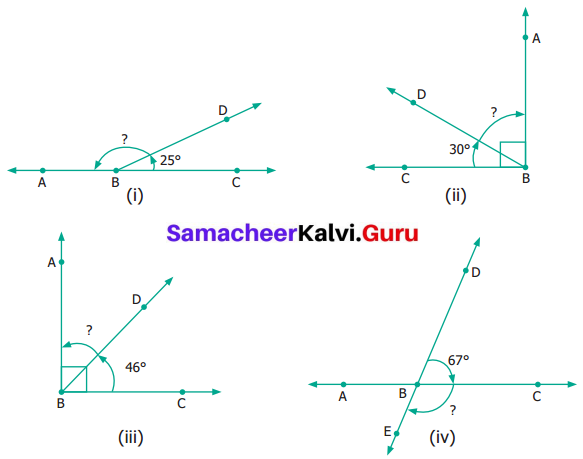Solution:
(i) If two angles add up to 180°, they are supplementary.
We know that the straight angle ∠CBC = 180°
Given ∠CBD = 25°
∴ ∠DBA = 180° – 25° = 155°
∴ Supplementary ∠DBA = 155°

(ii) If two angles add upto 90°, they are complementary
Given ∠ABC = 25°
∠CBD = 30°
∴ ∠DBA = 90° – 30° = 60°
∴ Complementary angle ∠DBA = 60°

(iii) Given ∠CBA = 90°
∠CBD = 46°
∴ ∠DBA = 90° – 46° = 44°
We know that the sum of two angles is 90°, then they are complementary
∴ Complementary angle ∠DBA = 44°

(iv) Given ∠DBE = 180°
∠DBC = 67°
∴ ∠CBE = 180° – 67° = 113°
Here ∠DBC + ∠DBE = 180°
∴ Supplementary angle ∠CBE = 113°Objective Type Questions

Question 11.
In this Figure, which is not the correct way of naming an angle?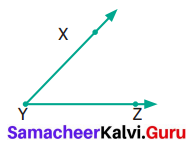(a) ∠Y
(b) ∠ZXY
(c) ∠ZYX
(d) ∠XYZ
Solution:
(b) ∠ZXY

Question 12.
In this Figure, ∠AYZ = 45°. If the point ‘A’ is shifted to point ‘B’ along the ray, then the measure of ∠BYZ is ____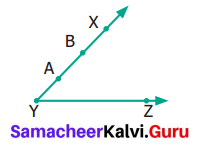(a) more than 45°
(b) 45°
(c) less than 45°
(d) 90°
Solution:
(b) 45°
Hint: ∠XYZ = ∠BYZ = ∠AYZ